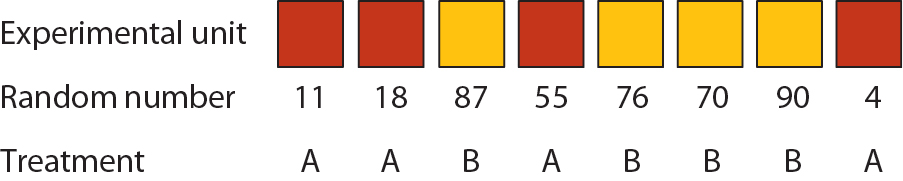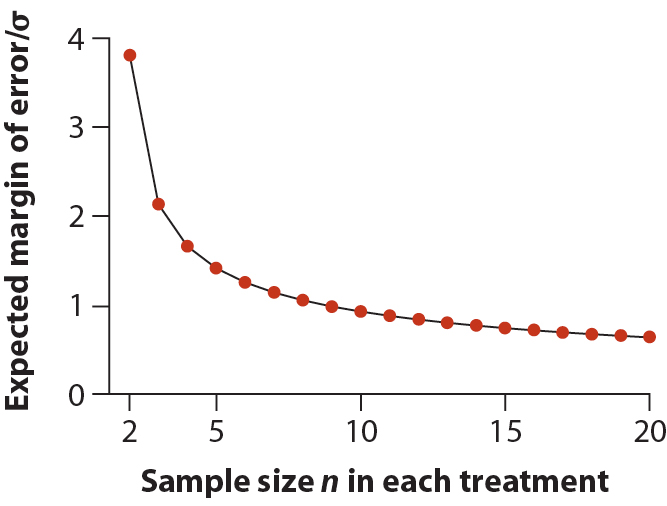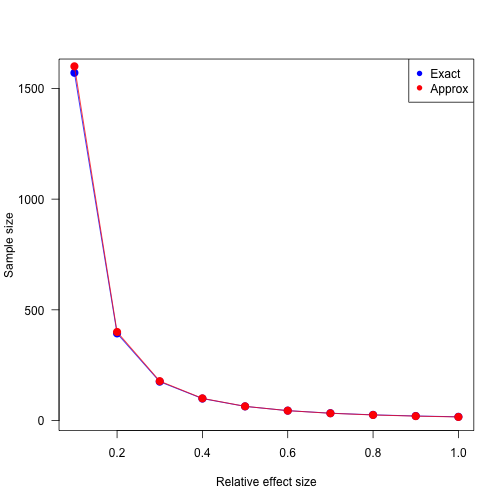# Between-individual variation, replication, and sampling (cont'd)

M. Drew LaMar
March 30, 2016

“There are no null results; there are only insufficiently clever choices of $$H_0$$. ”

- @richarddmorey

### Proper randomization

Assigning treatments to subjects (one possibility):1. List all $$n$$ subjects, one per row, in a spreadsheet.
2. Use the computer to give each subject a random number.
3. Assign treatment A or B to those subjects receiving the lowest or highest numbers, respectively.

### Randomization in time

Remember, randomization is important in all processes of the experiment, including preparation, setup, and measurement.

Randomize measurement of replicates in time:

• Watching 50 hours of great tit courtship behaviour on video increases your ability to observe
• After 10 hours of counting through a microscope, tiredeness kicks in
• Aging equipment

This shows time of measurement could be a confounding factor.

### Examples, examples, examplesBirdsongs and attractiveness

Question: How do we measure relationship between male birdsongs and attractiveness to females?

Experimental Design: Record the complex song of one male and the simple song of another male, and then play these same two songs to each of 40 different females. Compute a confidence interval for the mean attractiveness of the two male songs.

Discuss: What is wrong with this design so far?

Answer: Each measure of female choice is a pseudoreplicate ($$n=40$$).

### Examples, examples, examplesDiscuss: What is wrong with this design so far?

Answer: Each measure of female choice is a pseudoreplicate ($$n=40$$).

Discuss: What can we do to correct for this pseudoreplication?

Answer: Record songs of 40 males with complex songs, and 40 separate males with simple songs. Each female should listen to a unique pair of songs, one simple and one complex. Design can get even more complicated than this.

Discuss: What are examples of confounding variables in the pseudoreplicated case?

### Examples, examples, examplesBlood sugar levels

Experimental Design: Phlebotomist takes 15 samples from each of 10 patients, yielding a total of 150 measurements.

Discuss: What is the replicate and sample size in this situation? Why?

### Examples, examples, examplesAntibiotics and bacterial growth rates

Experimental Design: Two agar plates: one with antibiotic, one without. Spread bacteria on both plates, let them grow for 24 hours, then measure diameter of 100 colonies on each plate?

Discuss: What is the replicate and sample size in this situation? Why?

### What sample size should I use?

Three things:

• Plan for precision (estimation)
• Plan for power (hypothesis testing)
• Plan for data loss

We'll use a two-sample $$t$$-test as the example in this section.

### Plan for precision

We would like to compute a 95% confidence interval for $$\mu_{1}-\mu_{2}$$.

$\bar{Y}_{1}-\bar{Y}_{2} \pm \mathrm{margin \ of \ error},$

where “margin of error” is the half-width of the 95% confidence interval.

In this case, the following formula is an approximation to the number of samples needed to achieve the desired margin of error (assuming balanced design, i.e. $$n_{1}=n_{2}=n$$):

$n \approx 8\left(\frac{\mathrm{margin \ of \ error}}{\sigma}\right)^{-2}$

### Plan for precision### Plan for power

Two-sample $$t$$-test:

$H_{0}: \mu_{1} - \mu_{2} = 0.$ $H_{A}: \mu_{1} - \mu_{2} \neq 0.$

A conventional power to aim for is 0.80, i.e. we aim to prove $$H_{0}$$ is false in 80% of experiments.

Assuming a significance level of 0.05, a quick approximation to the planned sample size $$n$$ in each of two groups is

$n \approx 16\left(\frac{D}{\sigma}\right)^{-2},$

where $$D = |\mu_{1}-\mu_{2}|$$ is the effect size.

### Pwr package in R

library(pwr)

function power calculations for
pwr.2p.test two proportions (equal n)
pwr.2p2n.test two proportions (unequal n)
pwr.anova.test balanced one way ANOVA
pwr.chisq.test chi-square test
pwr.f2.test general linear model
pwr.p.test proportion (one sample)
pwr.r.test correlation
pwr.t.test t-tests (one sample, 2 sample, paired)
pwr.t2n.test t-test (two samples with unequal n)

### Two-sample t-test example

Two-sample $$t$$-test with significance level 0.05, 80% power, and relative effect size $$d = \frac{|\mu_{1}-\mu_{2}|}{\sigma} = 0.3$$.

pwr.t.test(d=0.3, power=0.8, type="two.sample")


Two-sample t test power calculation

n = 175.3847
d = 0.3
sig.level = 0.05
power = 0.8
alternative = two.sided

NOTE: n is number in *each* group


### Two-sample t-test example